Paparazzi UAS  v5.15_devel-230-gc96ce27 Paparazzi is a free software Unmanned Aircraft System.
air_data.c File Reference

Air Data interface. More...

#include "modules/air_data/air_data.h"
#include "subsystems/abi.h"
#include "math/pprz_isa.h"
#include "state.h"
#include "generated/airframe.h"
#include "subsystems/datalink/telemetry.h"Include dependency graph for air_data.c:

Go to the source code of this file.

## Macros

ABI binding for absolute pressure. More...

ABI binding for differential pressure. More...

ABI binding for temperature. More...

ABI binding for airspeed. More...

ABI binding for incidence angles. More...

#define AIR_DATA_TAS_FACTOR   1.0
Default factor to convert estimated airspeed (EAS) to true airspeed (TAS) More...

#define AIR_DATA_CALC_AIRSPEED   TRUE
Calculate Airspeed from differential pressure by default. More...

#define AIR_DATA_CALC_TAS_FACTOR   TRUE
Calculate tas_factor from temp and pressure by default. More...

#define AIR_DATA_CALC_AMSL_BARO   FALSE
Don't calculate AMSL from baro and QNH by default. More...

## Functions

static void pressure_abs_cb (uint8_t sender_id, uint32_t stamp, float pressure)

static void pressure_diff_cb (uint8_t sender_id, float pressure)

static void temperature_cb (uint8_t sender_id, float temp)

static void airspeed_cb (uint8_t sender_id, float eas)

static void incidence_cb (uint8_t sender_id, uint8_t flag, float aoa, float sideslip)

static void send_baro_raw (struct transport_tx *trans, struct link_device *dev)

static void send_air_data (struct transport_tx *trans, struct link_device *dev)

static void send_amsl (struct transport_tx *trans, struct link_device *dev)

void air_data_init (void)
AirData initialization. More...

float air_data_get_amsl (void)
Return AMSL (altitude AboveSeaLevel). More...

void air_data_periodic (void)
Check health. More...

float eas_from_dynamic_pressure (float q)
Calculate equivalent airspeed from dynamic pressure. More...

float get_tas_factor (float p, float t)
Calculate true airspeed (TAS) factor. More...

float tas_from_eas (float eas)
Calculate true airspeed from equivalent airspeed. More...

float tas_from_dynamic_pressure (float q)
Calculate true airspeed from dynamic pressure. More...

## Variables

struct AirData air_data
global AirData state More...

static abi_event pressure_abs_ev

static abi_event pressure_diff_ev

static abi_event temperature_ev

static abi_event airspeed_ev

static abi_event incidence_ev

static uint8_t baro_health_counter
counter to check baro health More...

## Detailed Description

Air Data interface.

• pressures
• airspeed
• angle of attack and sideslip
• wind

Definition in file air_data.c.

## Macro Definition Documentation

ABI binding for airspeed.

Definition at line 65 of file air_data.c.

Referenced by air_data_init().

ABI binding for absolute pressure.

Definition at line 44 of file air_data.c.

Referenced by air_data_init().

ABI binding for differential pressure.

Definition at line 51 of file air_data.c.

Referenced by air_data_init().

 #define AIR_DATA_CALC_AIRSPEED   TRUE

Calculate Airspeed from differential pressure by default.

Definition at line 83 of file air_data.c.

Referenced by air_data_init().

 #define AIR_DATA_CALC_AMSL_BARO   FALSE

Don't calculate AMSL from baro and QNH by default.

Definition at line 93 of file air_data.c.

Referenced by air_data_init().

 #define AIR_DATA_CALC_TAS_FACTOR   TRUE

Calculate tas_factor from temp and pressure by default.

Definition at line 88 of file air_data.c.

Referenced by air_data_init().

ABI binding for incidence angles.

Definition at line 72 of file air_data.c.

Referenced by air_data_init().

 #define AIR_DATA_TAS_FACTOR   1.0

Default factor to convert estimated airspeed (EAS) to true airspeed (TAS)

Definition at line 78 of file air_data.c.

Referenced by air_data_init().

ABI binding for temperature.

Definition at line 58 of file air_data.c.

Referenced by air_data_init().

## Function Documentation

 float air_data_get_amsl ( void )

Return AMSL (altitude AboveSeaLevel).

If AMSL from baro is valid, return that, otherwise from gps.

Definition at line 257 of file air_data.c.

Referenced by tas_from_eas().Here is the call graph for this function:Here is the caller graph for this function:

 void air_data_init ( void )

AirData initialization.

Called at startup. Bind ABI messages

Definition at line 216 of file air_data.c.Here is the call graph for this function:

 void air_data_periodic ( void )

Check health.

Needs to be called periodically.

Definition at line 267 of file air_data.c.

References air_data, and AirData::amsl_baro_valid.

 static void airspeed_cb ( uint8_t sender_id, float eas )
static

Definition at line 161 of file air_data.c.

Referenced by air_data_init().Here is the call graph for this function:Here is the caller graph for this function:

 float eas_from_dynamic_pressure ( float q )

Calculate equivalent airspeed from dynamic pressure.

Dynamic pressure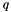(also called impact pressure) is the difference between total(pitot) and static pressure.

Airspeed from dynamic pressure: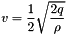with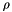as air density. Using standard sea level air density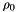gives you equivalent airspeed (EAS).

Parameters
 q dynamic pressure in Pa
Returns
equivalent airspeed in m/s

Definition at line 291 of file air_data.c.

References Max, and PPRZ_ISA_AIR_DENSITY.

Referenced by pressure_diff_cb(), and tas_from_dynamic_pressure().Here is the caller graph for this function:

 float get_tas_factor ( float p, float t )

Calculate true airspeed (TAS) factor.

TAS = tas_factor * EAS

True airspeed (TAS) from equivalent airspeed (EAS):and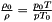where

•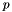is the air pressure at the flight condition
•is the air pressure at sea level = 101325 Pa
•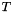is the air temperature at the flight condition
•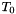is the air temperature at sea level = 288.15 K
Parameters
 p current air pressure in Pa t current air temperature in degrees Celcius
Returns
tas factor

Definition at line 319 of file air_data.c.

References KelvinOfCelsius, PPRZ_ISA_SEA_LEVEL_PRESSURE, and PPRZ_ISA_SEA_LEVEL_TEMP.

Referenced by tas_from_eas(), and temperature_cb().Here is the caller graph for this function:

 static void incidence_cb ( uint8_t sender_id, uint8_t flag, float aoa, float sideslip )
static

Definition at line 172 of file air_data.c.

Referenced by air_data_init().Here is the call graph for this function:Here is the caller graph for this function:

 static void pressure_abs_cb ( uint8_t sender_id, uint32_t stamp, float pressure )
static

FIXME: use height above MSL (geoid) and not WGS84 ellipsoid here

Definition at line 112 of file air_data.c.

Referenced by air_data_init().Here is the call graph for this function:Here is the caller graph for this function:

 static void pressure_diff_cb ( uint8_t sender_id, float pressure )
static

Definition at line 139 of file air_data.c.

Referenced by air_data_init().Here is the call graph for this function:Here is the caller graph for this function:

 static void send_air_data ( struct transport_tx * trans, struct link_device * dev )
static

Definition at line 195 of file air_data.c.

Referenced by air_data_init().Here is the caller graph for this function:

 static void send_amsl ( struct transport_tx * trans, struct link_device * dev )
static

Definition at line 204 of file air_data.c.

References air_data, LlaCoor_f::alt, AirData::amsl_baro, and stateGetPositionLla_f().

Referenced by air_data_init().Here is the call graph for this function:Here is the caller graph for this function:

 static void send_baro_raw ( struct transport_tx * trans, struct link_device * dev )
static

Definition at line 189 of file air_data.c.

References air_data, AirData::differential, and AirData::pressure.

Referenced by air_data_init().Here is the caller graph for this function:

 float tas_from_dynamic_pressure ( float q )

Calculate true airspeed from dynamic pressure.

Dynamic pressure(also called impact pressure) is the difference between total(pitot) and static pressure.

Parameters
 q dynamic pressure in Pa
Returns
true airspeed in m/s

Definition at line 365 of file air_data.c.

References eas_from_dynamic_pressure(), and tas_from_eas().

Referenced by pressure_diff_cb().Here is the call graph for this function:Here is the caller graph for this function:

 float tas_from_eas ( float eas )

Calculate true airspeed from equivalent airspeed.

True airspeed (TAS) from EAS: TAS = air_data.tas_factor * EAS

Parameters
 eas equivalent airspeed (EAS) in m/s
Returns
true airspeed in m/s

Definition at line 338 of file air_data.c.

Referenced by airspeed_cb(), pressure_diff_cb(), and tas_from_dynamic_pressure().Here is the call graph for this function:Here is the caller graph for this function:

 static void temperature_cb ( uint8_t sender_id, float temp )
static

Definition at line 151 of file air_data.c.

Referenced by air_data_init().Here is the call graph for this function:Here is the caller graph for this function:

## Variable Documentation

 struct AirData air_data

global AirData state

Definition at line 39 of file air_data.c.

 abi_event airspeed_ev
static

Definition at line 67 of file air_data.c.

 uint8_t baro_health_counter
static

counter to check baro health

Definition at line 109 of file air_data.c.

 abi_event incidence_ev
static

Definition at line 74 of file air_data.c.

 abi_event pressure_abs_ev
static

Definition at line 46 of file air_data.c.

 abi_event pressure_diff_ev
static

Definition at line 53 of file air_data.c.

 abi_event temperature_ev
static

Definition at line 60 of file air_data.c.